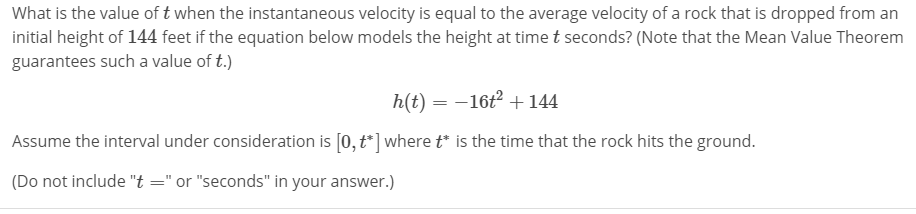What is the value of t when the instantaneous velocity is equal to the average velocity of a rock that is dropped from aninitial height of 144 feet if the equation below models the height at time t seconds? (Note that the Mean Value Theoremguarantees such a value of t.)h(t) --16+144Assume the interval under consideration is [0,t ] wheretis the time that the rock hits the ground.(Do not include "t"or "seconds" in your answer.)

Questionhelp_outlineImage TranscriptioncloseWhat is the value of t when the instantaneous velocity is equal to the average velocity of a rock that is dropped from an initial height of 144 feet if the equation below models the height at time t seconds? (Note that the Mean Value Theorem guarantees such a value of t.) h(t) --16+144 Assume the interval under consideration is [0,t ] wheretis the time that the rock hits the ground. (Do not include "t"or "seconds" in your answer.) fullscreen
Step 1

To calculate the value of "t" referred to in the problem

Step 2

first determinet t* (the time taken to hit the ground)

Step 3

Using t*=3, calculate the av...

Want to see the full answer?

See Solution

Want to see this answer and more?

Our solutions are written by experts, many with advanced degrees, and available 24/7

See Solution
Tagged in

Calculus